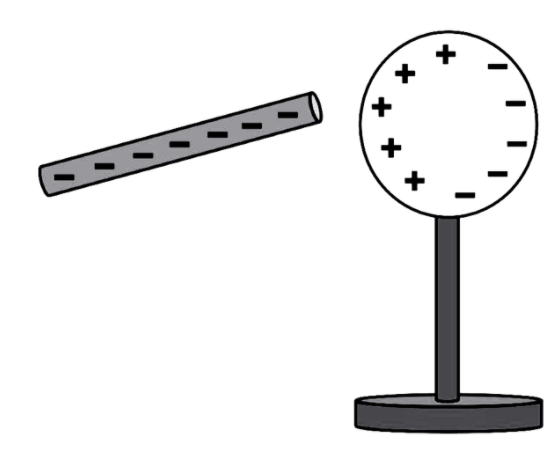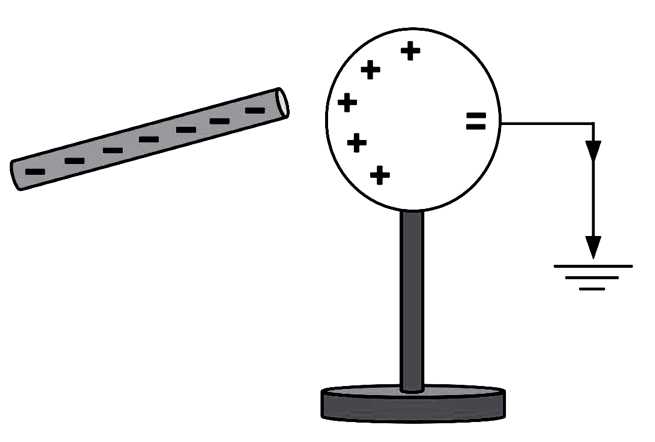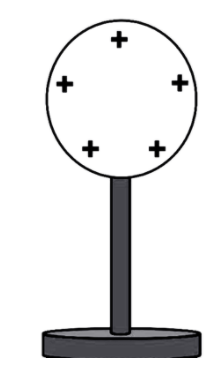Home/Class 12/Physics/

## QuestionPhysicsClass 12

How can you charge a metal sphere positively without touching ?

By electrostatic induction
4.64.6## Solution

Our aim is to charge a metal sphere positively without touching it. This can be achieved by means of electrostatic induction.
$$1.$$ A negatively charged rod is brought near a metallic sphere kept on an insulating stand to charge it as shown in  $$\mathit{Fig.}(a)$$ . Since the rod is full of negative charges alone, due to attraction, the positive charges of metal sphere get attracted by the rod and will get concentrated near it. The negative charges of the sphere move away to the farther end due to repulsion. And the nearer end of the sphere becomes positively charged due to the shortfall of electrons.Figure $$(a)$$
$$2.$$ Then connect the sphere to the ground using a conducting wire as shown in  $$\mathit{Fig.}(b).$$  The free electrons will start to flow into the ground from the sphere.Figure$$(b)$$
$$3.$$ Now disconnect the rod and we can observe the uniform spread of positive charges over the sphere as shown in  $$\mathit{Fig.}(c)$$ .Figure$$(c)$$
Thus, charging a metal sphere positively is achieved without touching it by means of induction.